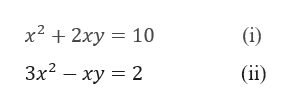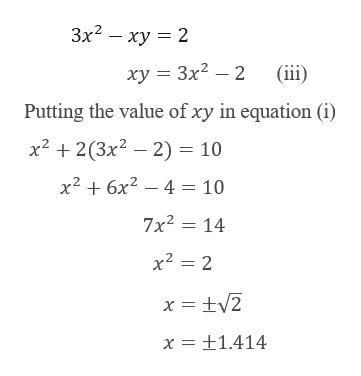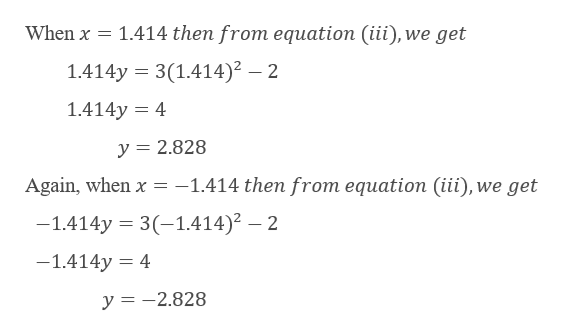X2+2xy=103x2 -xy=2Solve systems of nonlinear equations and graphing

Question

X2+2xy=10

3x2 -xy=2

Solve systems of nonlinear equations and graphing

Step 1

Given,help_outlineImage Transcriptioncloseх? + 2ху 3D 10 (i) Зx2 — ху %3D 2 (ii) fullscreen
Step 2

Now, from equation (ii), we gethelp_outlineImage TranscriptioncloseЗx2 — ху — 2 (iii) ху 3 Зx2 — 2 Putting the value of xy in equation (i x2 2(3x2 2)= 10 x26x24 = 10 7x2 14 x2 2 x +1.414 fullscreen
Step 3

Now,

...help_outlineImage Transcriptionclose1.414 then from equation (iii), we get When x 1.414y 3(1.414)2 2 1.414y 4 y =2.828 -1.414 then from equation (iii),we get Again, when x -1.414y 3(-1.414)2 - 2 -1.414y 4 y =-2.828 fullscreen

Want to see the full answer?

See Solution

Want to see this answer and more?

Our solutions are written by experts, many with advanced degrees, and available 24/7

See Solution
Tagged in

Other